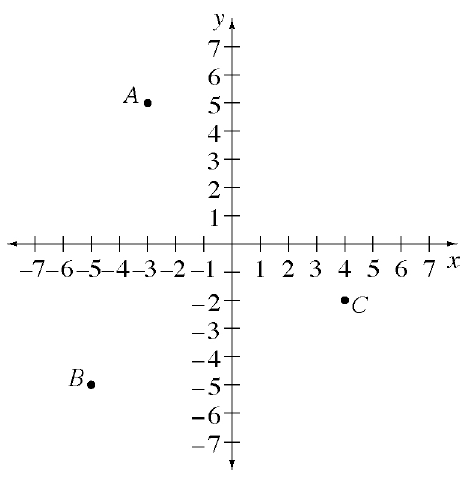### Home > CC2MN > Chapter 2 > Lesson 2.2.1 > Problem2-40

2-40.

One of the topics you will review in this course is reading graphs.  Look at the graph at right.  This graph shows positive and negative values on both axes.  It divides the flat surface into four parts, or quadrants, and is therefore called a four-quadrant graph.

1. The coordinates (the $x\text{‑}$ and $y\text{‑values}$) for point $A$ are $(-3,5)$.  Explain how these numbers tell you the position of point $A$ using the graph.

Remember: The first number in the ordered pair tells you the number of units left or right from the origin.
What does the second number in the ordered pair tell you?

2. Name the coordinates $(x,y)$ for points $B$ and $C$.

3. If Samantha moved point $A$ $9$ units down and $6$ units to the right, at what point would she end up?